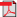## RIEB Discussion Paper Series No.2016-08### Title

Recursive Utility and the Solution to the Bellman Equation

### Abstract

This study infinite-horizon deterministic dynamic programming problems based on recursive utility in discrete time. Under a small number of conditions, we show that the Bellman operator has a fixed point using Knaster-Tarski's fixed point theorem. We also show the fixed point of the Bellman operator can be computed by iteration from the initial function between the lower boundary and the fixed point. To show the convergence theorem, we use Tarski-Kantorovitch's fixed point theorem.

### Keywords

Recursive utility, Fixed point theorem, Dynamic programming, Bellman equation

C61, O41

### Inquiries

Masayuki YAO
Research Associate (Non-tenured), Department of Economics, Keio University,
2-15-45 Mita, Minato-ku, Tokyo, 108-8345 Japan